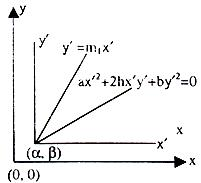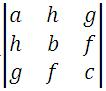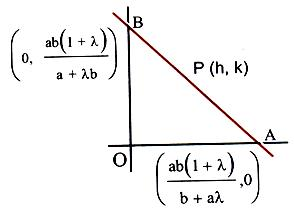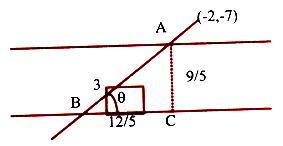×#### Thank you for registering.

One of our academic counsellors will contact you within 1 working day.

Click to Chat

1800-1023-196

+91-120-4616500

CART 0

• 0

MY CART (5)

Use Coupon: CART20 and get 20% off on all online Study Material

ITEM
DETAILS
MRP
DISCOUNT
FINAL PRICE
Total Price: Rs.

There are no items in this cart.
Continue Shopping```Family of Lines

Table of Content

What do you mean by a pair of Straight Lines?

General Equation of Second Degree representing a Pair of Straight Lines

What do you mean by the joint equation of a pair of Straight Lines?

What is family of lines?

Related ResourcesStraight lines is an important topic of mathematics syllabus of IIT JEE. The topic is quite vast and requires thorough understanding of the concepts. When we talk of straight lines, other topics that come to our mind are pair of straight lines and family of lines. This section stresses on these topics in detail.

Before we proceed toward family of straight lines we shall first discuss pair of straight lines and the joint equation of pair of straight lines.

What do you mean by a pair of Straight Lines?

An equation of the type ax2 + 2hxy + by2 which is a homogeneous equation of degree 2 denotes a pair of straight lines passing through the origin. The lines may be real, coincident or imaginary depending on the conditions satisfied by them:

If h2 > ab, then the lines are real and distinct.

If h2 < ab, then the lines are imaginary with the point of intersection as (0, 0).

If h2 = ab, then the lines are coincident.

If α is the acute angle between the pair of straight lines, then tan α = |2√(h2-ab)/(a + b)|.

These lines are perpendicular to each other if the coefficient of x2 + coefficient of y2 = 0.

If the coefficient of xy = 0, then the lines are equally inclined to the x-axis.

The lines are said to be coincident if h2 = ab.

Now, we throw some light on the General Equation of Second Degree representing a Pair of Straight Lines

The equation ax2 + 2hxy + by2 + 2gx + 2fy + c = 0 represents a second degree equation where a, h, b don’t vanish simultaneously.

Let a ≠ 0.

Now, the above equation becomes

a2x2 + 2ax (hy + g) = aby2 – 2afy – ac

On completing the square on the left side, we get,

a2x2 + 2ax (hy + g) = y2(h2 – ab) + 2y(gh – af) + g2 – ac.

i.e. (ax + hy + g) = + √y2(h2–ab) + 2y(gh–af)g2 – ac

We cannot obtain x in terms of y, involving only terms of the first degree, unless the quantity under the radical sign be a perfect square. The condition for this is,

(gh – af)2 = (h2 – ab) (g2 – ac)

i.e. g2h2 – 2afgh + a2f2 = g2h2 – abg2 – abg2 – ach2 + a2bc

Cancelling and dividing by a, we have the required condition as

abc + 2fgh – af2 – bg2 – ch2 = 0

Note:

The angle α between two lines represented by the general equation is the same as the one represented by its homogeneous part.

Illustration 1:

What is the point of intersection of two straight lines given by general equation ax2 + 2hxy + by2 + 2gx + 2fy + c = 0?

Solution:

The general solution is

ax2 + 2hxy + by2 + 2gx + 2fy + c = 0                   …… (1)

Let (α, ß) be the point of intersection we consider line paralleled transformation.

x = x’ + α,         y = y’ + ß

From (1) we have

a(x’ + α)2 + 2h(x’ + α) (y’ + ß) + b(y’ + ß)2 + 2g(x’ + α) + 2f(y’ + ß) + c = 0

⇒ ax’2 + 2hx’y’ + by’2 + aα2 + 2hαß + bß2 + 2gα + 2fß + 2x’(a α + hß + g) + 2y’ + 2y’ (hα + bß + f) = 0

⇒ ax’2 + 2hx’y’ + by’2 + 2x’(aα + hß + g) + 2g’ + 2y’ (hα + bß + f) = 0Which must be in the form ax'2 + 2hx’y’ + by’ = 0

This cannot be possible unless aα + hß + g = 0

hα + bß + f = 0

Solving α/hf–bg = ß/hg–af = 1/ab–h2

α = hf–bg/ab–b2, ß = hg–af/ab–h2

Illustration 2:

Represent lines parallel to y = 2x and y = 3x by a second degree equation

Solution:

The lines parallel to y = 2x and y = 3x can be represented as

(y – 2x – c1) (y – 3x – c2) (where c1 and c2 are constants)

This gives 6x2 – 5xy + y2 + (3c1 + 2c2) x + (– c1 – c2) y + c1 c2 = 0

Note:

1. Homogeneous part is same as for the equation of above illustration. Therefore, the homogeneous part of a general second degree equation determines the slope of the lines i.e. lines parallel to ax2 + 2hxy + by2 + c = 0 and through the origin are represented by the equation ax2 + 2hxy + by2 = 0

2. The equation ax2 + 2hxy + by2 + 2fy + c = 0 represents a pair of parallel straight lines if h/a = b/h = f/g or bg2 = af2

The distance between them is given by 2√g2–ac/a(a+b) or √f2–bc/b(a+b).

Illustration 3:

Does the second degree equation x2 + 3xy + 2y2 – x – 4y – 6 = 0 represent a pair of lines? If yes, find their point of intersection.

Solution:

We observe that a = 1, h = 3/2, b = 2, g = –1/2, f = 2, c = – 6

abc + 2fgh – af2 – bg2 – ch2 = – 12 + 3 – 4 – 1/2 + 27/2 = 0

Therefore the given second-degree equation represents a pair of lines, x2 + 3xy + 2y2 – x – 4y – 6 = (x + 2y + 2) (x + y – 3).

Consider the equations formed by first two rows of.

i.e. ax + hy + g = 0 and hx + by + f = 0

i.e. x + 3/2 y – 1/2 and 3/2 x + 2y – 2 = 0

Solving these, we get the required point of intersection.

i.e. 2x + 3y – 1 = 0

3x – 4y – 4 = 0

Solving the above equation, we get x = 8, y = –5.

What do you mean by the joint equation of a pair of Straight Lines?

If the line lx + my + n = 0, (n ≠ 0) i.e. the line not passing through origin) cuts the curve ax2 + by2 + 2gx + 2fy + c = 0 at two points A and B, then the joint equation of straight lines passing through A and B and the origin is given by homogenizing the equation of the curve by the equation of the line. i.e. if we have a line lx + my + n = 0 and a second degree curve given by the equation ax2 + 2hxy + by2 + 2gx + 2fy + c = 0, then the joint equation of a pair of straight lines joining origin to the points of intersection of both is given byax2 + 2hxy + by2 + (2gx + 2fy) (lx+my/–n) + c (lx+my/–n)2 = 0. This is the equation of the lines OA and OB.

Illustration 1:

Prove that the straight lines joining the origin to the points of intersection of the straight line hx + ky = 2hk and the curve (x – k)2 + (y – y)2 = c2 are at right angles if h2 + k2 = c2.

Solution:

Making the equation of the curve homogeneous with the help of that of the line, we get

x2 + y2 –2(kx + hy) (hx+ky/2hk) + (h2 + k2 – c2) (hx+ky/2hk) = 0

or 4h2k2x2 + 4h2k2y2 – 4h2x(hx+ky) – 4h2ky(hx + ky) + (h2 + k2 – c)(h2x2 + k2y2 + 2hxy) = 0.

This is the equation of the pair of lines joining the origin to the points of intersection of the given line and the curve. They will be at right angles if

Coefficient of x2 + coefficient of y2 = 0 i.e.

(h2 + k2) (h2 + k2 – c2) = 0 ⇒ h2 + k2 = c2       (since h2 + k2 ≠ 0).

What is family of lines?

Having studied pair of lines and the joint equation of pair of lines, we now proceed toward the family of lines. The general equation of the family of lines through the point of intersection of two given lines is L + λL’ = 0, where L = 0 and L’ = 0 are the two given lines, and λ is a parameter.

Remark:

1. Conversely, a line of the form L1 + λL2 = 0 passes through the point which is the point of intersection of the lines L1 = 0 and L2 = 0.

2. This also implies that if a linear expression contains an undetermined coefficient then that represents a family of straight lines. Note that in this case, the line L= 0 cannot be a fixed line.

3. If the lines L1 and L2 are parallel lines then they meet at infinity.

4. If we have a line ax + by + c = 0, then the line perpendicular to this line is given by bx - ay + k = 0, where k is a parameter.

5. If we have a line ax + by + c = 0, then the line parallel to this line is given by ax + by + k = 0, where k is a parameter.

Illustration 1:

A variable line through the point of intersection of the lines x/a + y/b = 1 and x/b + y/a = 1 meets the coordinate axes in A and B. Show that the locus of the midpoint of AB is the curve 2xy(a + b) = ab(x + y).

Solution:

Let (h, k) be the midpoint of the variable line AB.

The equation of the variable line AB is (bx + ay – ab) + λ(ax + by – ab) = 0Coordinates of A are (ab(1+λ)b + λa).

Coordinate of B are (0, ab(1+λ)b + λa).

Mid-point of AB is (ab(1 + λ)2(b + λa), ab(1 + λ)2(a + λb))

⇒ h = ab(1+λ)2(b+λa); k = ab(1+λ)2(a+λb)

⇒ 1/2h = b+λa/ab(1+λ); 1/2k = a+λb/ab(1+λ)

⇒ 1/2h + 1/2k = a+b/ab ⇒ (h + k)ab = 2hk (a + b).

Hence the locus of the midpoint of AB is (x + y) ab = 2xy (a + b).

To find the equation to the straight lines which pass through a given point (x1, y1) and make equal angles with the given straight line y = m1x + c.

If m is the slope of the required line and α is the angle which this line makes with the given line, then tan a = + (m1–m)/(1+m1m).1. The above expression for tan α, gives two values of m, say mA and mB.

2. The required equations of the lines through the point (x1, y1) and making equal angles α with the given line are y – y1 = mA (x – x1), y – y1 = mB(x – x1).

Illustration 1:

Find the equations to the sides of an isosceles right-angled triangle, the equation of whose hypotenuse is 3x + 4y = 4 and the opposite vertex is the point (2, 2).

Solution:

The problem can be restated as:

Find the equations to the straight lines passing through the given point (2, 2) and making equal angles of 45o with the given straight line 3x + 4y – 4 = 0

Slope of the line 3x + 4y – 4 = 0 is m1 = –3/4⇒ tan 45o = + m1–m/1+m1m, i.e., 1 = + m+3/4/1–3/4m

So that mA = 1/7 and mB = –7.

Hence the required equations of the two lines are

y – 2 = mA (x – 2) and y – 2 = mB(x – 2).

⇒ 7y – x – 12 = 0 and 7x + y = 16.

Illustration 2:

The straight lines 3x + 4y = 5 and 4x – 3y = 15 intersect at the point A. On these lines points B and C are chosen so that AB = AC. Find the possible equations of the line BC passing through (1, 2).

Solution:

The two given straight lines are at right angles.

Since AB = AC, the triangle is an isosceles right angled triangle.

The required equation is of the form y – 2 = m(x – 1)              … (1)

with tan 45o = + m+3/4/1–3m/4 = + m–4/3/1+4m/3

⇒ 1 = + m+3/4/1–3m/4 = + m–4/3/1+4m/3 ⇒ m = – 7, 1/7.

Substitute the value of m in (1). We get the required equations.

Illustration 3:

Find the equation of the straight line passing through (–2, 7) and having intercept of length 3 units between the straight lines 4x + 3y = 12 and 4x + 3y = 3.

Solution:

Distance AC between the two given parallel lines

= |c1–c2/√a2 + b2| = 12–3/√(16 + 9) = 9/5.Let AB be the intercept of length 3 units.

⇒ BC = 12/5. If θ is the angle between BC and AB, then tan θ = 9/12 = 3/4.

Slope of the parallel lines = –4/3 = m2.

If m1 is the slope of the required line, then tan θ = m1–m/1+m1m ⇒ 3/4 = + m1–4/3/1+4/3m1

i.e. m1 + 4/3 = 3/4 (1 – 4/3 m1) and m1 + 4/3 = 3/4 (1 – 4/3 m1).

The slopes are (i) m1 = –7/24 (ii) m1 = ∞ (the line is parallel to the y-axis).

The required equations of the lines are 7x + 24y + 182 = 0 and x + 2 = 0.

Alternative Solution:

Equation of the line, through P(–2, 7) and making angle θ with the x-axis, is x+2/cosθ = y+7/sin θ = r.

If this line intersects the given lines at A and B, with AB = 3, the points A and B are A(–2 + r1 cos θ, – 7 + r1 sin θ) and B (–2 + (r1 + 3) cos θ, – 7 + (r1 + 3) sin θ).

Since A and B lie on the lines 4x + 3y = 3 and 4x + 3y = 12, we have

4r1 cos θ + 3r1 sin θ = 32 and

4r1 cos θ + 3r1 sin θ + 12 cos θ + 9 sin θ = 41, so that

12 cos θ + 9 sin θ = 0 or 4 cos θ + 3 sin θ = 3.

Solving this equation we find that θ = π/2 and tan θ = –7/24.

Hence the required lines are x + 2 = 0 and y + 7 = –7/24 (x + 2) i.e. 7x + 24y + 182 = 0.

Related Resources

For getting an idea of the type of questions asked, refer the previous year papers.

To read more, Buy study materials of Straight Lines comprising study notes, revision notes, video lectures, previous year solved questions etc. Also browse for more study materials on Mathematics here.
```### Course Features

• 731 Video Lectures
• Revision Notes
• Previous Year Papers
• Mind Map
• Study Planner
• NCERT Solutions
• Discussion Forum
• Test paper with Video Solution